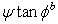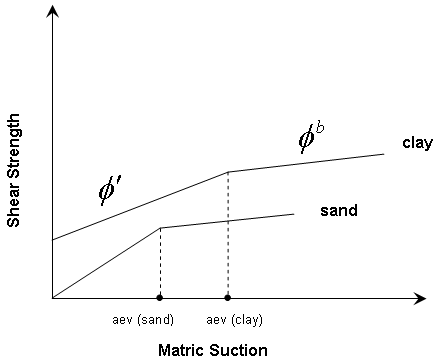The Rocscience International Conference 2021 Proceedings are now available. Read Now

# Unsaturated Shear Strength

The Unsaturated Shear Strength option in the Define Material Properties dialog is only enabled if:

A separate negative pore pressure cut off value is also added into the project setting. The pore pressure that used to calculate the unsaturated strength will be cut off at the input value.

After a seepage analysis has been computed in RS2, the resulting pore pressure distribution may indicate negative pore pressures (commonly referred to as matric suction), in the unsaturated zone above the water table. It is known that matric suction can contribute to the shear strength of a soil.

## Unsaturated Shear Strength Angle (Phi_b)

By specifying an Unsaturated Shear Strength Angle (Phi_b) for a material, the shear strength of the material in the unsaturated zone will be INCREASED by the amount:By default, the Unsaturated Shear Strength Angle = 0. This means that matric suction in the unsaturated zone, WILL NOT have any effect on the shear strength of a material.

The Unsaturated Shear Strength Angle is usually not a well known quantity. To obtain an appreciation of its importance, a parametric study can be carried out, in which the Unsaturated Shear Strength Angle is varied between 0 and the Friction Angle of the material.

## Air Entry Value

Air Entry Value is defined in Fredlund and Rahardjo (1993) as the matric suction value that must be exceeded before air recedes into the soil pores. The Air Entry Value is also referred to as the "displacement pressure" in petroleum engineering or the "bubbling pressure" in ceramics engineering.

In terms of strength, it is the point at which the strength of the soil deviates from its saturated effective Mohr-Coulomb behavior. If matric suction exceeds the air entry value, the strength is assumed to follow a linear envelope with Phi_b as the friction angle. Thus RS2 uses a bilinear strength envelope where:

• c' and phi' are used with positive pore pressures, and with negative pore pressures (matric suction) up to the air entry value
• Phi_b is used when negative pore pressure exceeds the air entry value.

The following figure (after Fredlund (2000) ) illustrates the effect of the air entry value. For matric suction less than the air entry value, the saturated effective stress friction angle is used. For matric suction greater than the air entry value, the unsaturated shear strength angle, Phi_b is used.

Effect of air entry value (aev) on unsaturated shear strength envelopes.